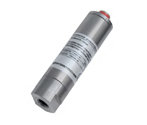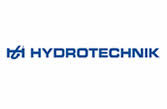## SensorsSensors Hydrotechnik

Sensors for different measurement categories

The success of a measure depends on the reliability of the data collection. The HySense® sensors by Hydrotechnik stand for quality and reliability. They are available for diverse measuring ranges, e.g. pressure, temperature, flow and other variables.Abbreviations for Product Names:

The two first letters at the beginning of the sensor’s name explains the measurement category:

PR = pressure

TE = Temperature

QT = Q= formula symbol of flow, T = Turbine

QG = Q= formula symbol of flow, G = Gear Flow Meter

QO = Q = formula symbol of flow, O from the Oval wheel

QL = Q = formula symbol of flow, L= Load

PS = P= Pressure, S = Switch

RS = R= Rotation Speed

TQ = Torque

TP = T= Temperatur, P= Pressure

PO = Position